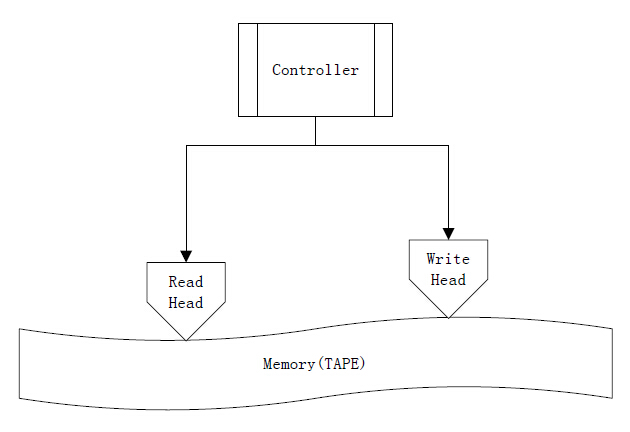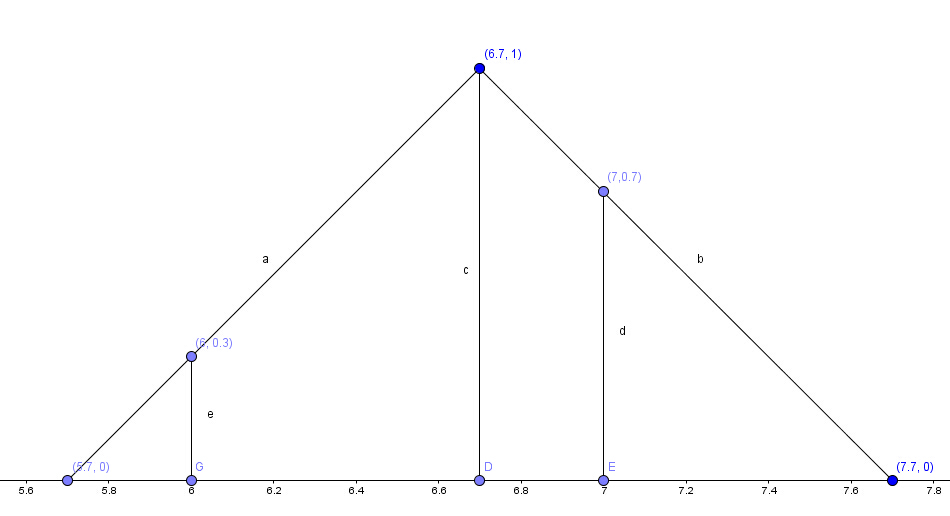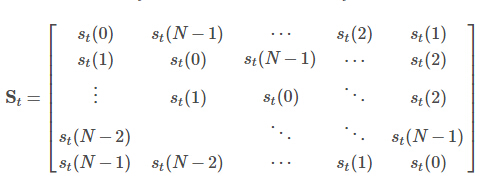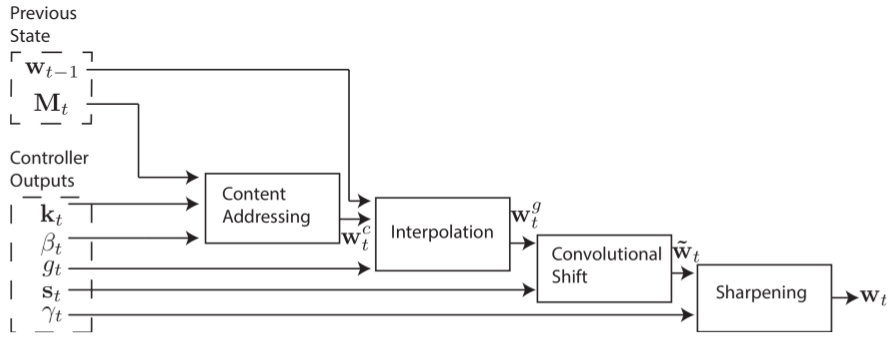# Neural Turing Machines-NTM系列（一）简述

NTM是一种使用Neural Network为基础来实现传统图灵机的理论计算模型。利用该模型，可以通过训练的方式让系统“学会”具有时序关联的任务流。

ppt:http://llcao.net/cu-deeplearning15/presentation/NeuralTuringMachines.pdf

GO语言实现：https://github.com/fumin/ntm

## 1.图灵机

TAPE：磁带，即记忆体（Memory）
register：状态寄存器，存储机器当前的状态。## 2. 神经图灵机（NTM）

iwt(i)=1,i,0wt(i)1 $\sum\limits_i w_t(i)=1,且\forall i,0≤w_t(i)≤1$

rt=iwt(i)Mt(i) $r_t=\sum\limits_i w_t(i)M_t(i)$

### 2.2 Writing 操作

1.擦除（erase）
wt $w_t$为Write Head发出的权值向量， et $e_t$为擦除向量，它们的所有分量值都在0,1之间，前一个时刻的Memory修改量为:
M˜t(i)=Mt1(i)[1wt(i)et] $\widetilde{M}_{t}(i)=M_{t-1}(i)\circ[1-w_t(i)e_t]$

Mt1=(142536) $M_{t-1}=\begin{equation} %开始数学环境 \left( %左括号 \begin{array}{ccc} %该矩阵一共3列，每一列都居中放置 1 & 2 & 3\\ %第一行元素 4 & 5 & 6\\ %第二行元素 \end{array} \right) %右括号 \end{equation}$
wt=[0.1,0.3,0.7]T $w_t=[0.1,0.3,0.7]^T$
et=[0.2,0.5,0.6] $e_t=[0.2,0.5,0.6]$
M˜t(1)=Mt1(1)[1wt(1)et] $\widetilde{M}_{t}(1)=M_{t-1}(1)\circ[1-w_t(1)e_t]$
=[1,2,3](10.1[0.2,0.5,0.6])=[1,2,3][0.98,0.95,0.94]=[0.98,1.9,1.88] $=[1,2,3]\circ(1-0.1*[0.2,0.5,0.6])=[1,2,3]\circ[0.98,0.95,0.94]=[0.98,1.9,1.88]$

Write Head还需要生成一个长度为 M $M$add$add$向量 at $a_t$，在 erase $erase$操作执行完之后，它将被“加”到对应的内存地址中。
t时刻的内存值将为：
Mt(i)=M˜t(i)+wt(i)at $M_t(i)=\widetilde{M}_{t}(i)+w_t(i)a_t$

## 3.NTM的寻址策略

### 3.1 Content-base(基于内容的寻址)：

wct(i)=eβtK[kt,Mt(i)]jeβtK[kt,Mt(j)] $w_t^c(i)=\frac {e^{\beta_tK[k_t,M_t(i)]}} {\sum\limits_je^{\beta_tK[k_t,M_t(j)]}}$

### 3.2 Location-base(基于位置的寻址)：

wgt=gtwct+(1gt)wt1 $w_t^g=g_tw_t^c+(1-g_t)w_{t-1}$st$s_t$生成之后，接下来就要使用 st $s_t$ wgt $w_t^g$进行循环卷积操作，具体如下式：
w˜t(i)=j=0N1wgt(j)st(ij) $\widetilde{w}_t(i)=\sum\limits_{j=0}^{N-1}w_t^g(j)s_t(i-j)$wt(i)=w˜t(i)γtjw˜t(j)γt $w_t(i)=\frac{\widetilde{w}_t(i)^{\gamma_t}}{\sum_j \widetilde{w}_t(j)^{\gamma_t}}$

St=st(0)st(1)st(2)st(3)st(4)st(4)st(0)st(1)st(2)st(3)st(3)st(4)st(0)st(1)st(2)st(2)st(3)st(4)st(0)st(1)st(1)st(2)st(3)st(4)st(0)=0.10.80.10000.10.80.10000.10.80.10.1000.10.80.80.1000.1 $\textbf{S}_t = \left[ \begin{array}{ccc} s_t(0) &s_t(4) & s_t(3) & s_t(2) & s_t(1)\\ s_t(1) &s_t(0) & s_t(4) & s_t(3) & s_t(2)\\ s_t(2) &s_t(1) & s_t(0) & s_t(4) & s_t(3)\\ s_t(3) &s_t(2) & s_t(1) & s_t(0) & s_t(4)\\ s_t(4) &s_t(3) & s_t(2) & s_t(1) & s_t(0)\\ \end{array} \right]=\left[ \begin{array}{ccc} 0.1&0 & 0 & 0.1 &0.8\\ 0.8 &0.1 & 0 & 0 & 0.1\\ 0.1 &0.8 & 0.1 & 0 & 0\\ 0 &0.1 & 0.8 & 0.1 & 0\\ 0 &0 & 0.1 & 0.8 & 0.1\\ \end{array} \right]$

w˜t=Stwgt=0.10.80.10000.10.80.10000.10.80.10.1000.10.80.80.1000.1×0.060.10.650.150.04=0.0530.0620.1510.5450.189 $\widetilde{\textbf{w}}_t=\textbf{S}_t \textbf{w}_t^g= \left[ \begin{array}{ccc} 0.1&0 & 0 & 0.1 &0.8\\ 0.8 &0.1 & 0 & 0 & 0.1\\ 0.1 &0.8 & 0.1 & 0 & 0\\ 0 &0.1 & 0.8 & 0.1 & 0\\ 0 &0 & 0.1 & 0.8 & 0.1\\ \end{array} \right] \times \left[ \begin{array}{ccc} 0.06\\ 0.1\\ 0.65\\ 0.15\\ 0.04\\ \end{array} \right]=\left[ \begin{array}{ccc} 0.053\\ 0.062\\ 0.151\\ 0.545\\ 0.189\\ \end{array} \right]$
γt=2 $\gamma_t=2$
wt=w˜γttjw˜t(j)γt=0.00780.01060.06300.82010.0986 $\textbf{w}_t=\frac{\widetilde{\textbf{w}}_t^{\gamma_t}}{\sum_j \widetilde{w}_t(j)^{\gamma_t}}=\left[ \begin{array}{ccc} 0.0078\\ 0.0106\\ 0.0630\\ 0.8201\\ 0.0986\\ \end{array} \right]$1.直接通过内容寻址，即前边提到的Content-base方式；
NTM的结构中存在很多的自由参数，比如内存的大小，读写头的数目，内存读取时的位移的范围。但是最重要的部分还是控制器的神经网络结构。比如，是选用递归网络还是前馈网络。如果选用LSTM，则其自有的隐层状态可以作为内存矩阵 Mt $M_t$的补充。如果把Controller与传统计算机的CPU进行类比，则 Mt $M_t$就相当于传统计算机的内存（RAM），递归网络中的隐层状态就相当于寄存器（Registers）,允许Controller混合跨越多个时间步的信息。另一方面，前馈网络可以通过在不同的时刻读取内存中相同的位置来模拟递归网络的特性。此外，基于前馈网络的Controller网络操作更为透明，因为此时的读写操作相比RNN的内部状态更容易解释。当然前馈网络的局限性主要在于同时存在的读写头数目有限。单一的Read Head每个时间步只能操作一个内存向量，而递归Controller则可通过内部存储器同时读取多个内存向量。